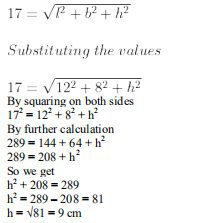# ML Aggarwal Solutions Class 9 Mathematics Solutions for Mensuration Exercise 16.4 in Chapter 16 - Mensuration

The length of the longest rod which can be kept inside a rectangular box is 17 cm. If the inner length and

the breadth of the box are 12 cm and 8 cm respectively, find its inner height.

Consider h m as the inner height

It is given that

Length of longest rod inside a rectangular box = 17 cm which is the same as the diagonal of a rectangular boxVideo transcript
"hello and welcome idea students i am rishabh karana the math you don't know leader and i'm here the new question it is the length of the longest rod which can be kept inside the rectangular boxes 17 centimeter if the inner length and the breadth of the box are 12 centimeter and eight individual respectively find it in a height so without wasting much time let's have a look at the solution so let's edge be the inner height and we have been given that length of the longest rod inside the rectangular boxes is equals to 17 centimeter which is nothing but the same as the diagonal of the rectangular box so now let's calculate the height and for that we'll use we'll write 17 is equals to root over l square plus b square plus h square which is nothing but the formula of the formula to find the diagonal of the rectangle the rectangular box so we'll substitute the values we get 17 is equal to the length is given as 12 so we'll write 12 square plus 8 square plus h square solving it further we get seventeen square to l square plus eight square plus h square so 289 minus 144 minus 64 is equals to h square so 17 square is 289 12 square is 144 and 8 square is 64. taking them taking the 12 square and the 8 square on the left hand side we get 289 minus 144 minus 64 which is nothing but equals to 81. that means that h is equals to root of 81 which means that as h is equals to 9 centimeter that means the inner height of the rectangular box is 9 centimeter so i hope my students got this question well don't forget to hit the subscribe button you can also share your doubts in the comment section see you next time bye"
Related Questions

Lido

Courses

Teachers

Book a Demo with us

Syllabus

Maths
CBSE
Maths
ICSE
Science
CBSE

Science
ICSE
English
CBSE
English
ICSE
Coding

Terms & Policies

Selina Question Bank

Maths
Physics
Biology

Allied Question Bank

Chemistry
Connect with us on social media!